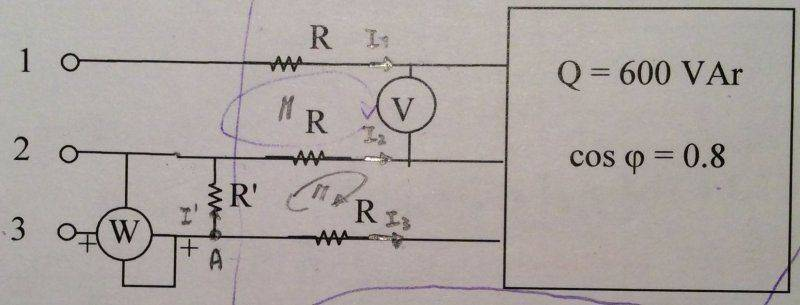# Evaluating indication of the wattmeter in three-phase system

pizzico85
Member warned about posting without the HW template
Hi all, I am new to this forum and I hope you can help me.

I have the following three-phase system where I have to evaluate the indication of the wattmeter W. Available data is: V = 380 V, R = 12 Ohm, R' = 120 Ohm.Can you make me understand the process for obtaining W?

## Answers and Replies

Staff Emeritus
You have 5 energy sinks here. Three identical resistors, one in each phase---that's fortunate, it maintains balance! There's a fourth resistor, and a 0.8pf load of Q=600VAr .

It looks like the wattmeter measures the power in R', in addition to the power in one phase of a balanced load.

What are your thoughts?

pizzico85
My thoughts are that I don't know how to calculate V' or I'.

I am completely in trouble.

I would like to understand how to proceed step by step to solve the problem.

Last edited:
Staff Emeritus
Since you know V, you should be able to calculate the line current going into that box on the right.

pizzico85
Since you know V, you should be able to calculate the line current going into that box on the right.

OK, the line current going into the box should be the following:

Since
Q = sqrt(3) * V * I * sin(phi)
cos(phi)
= 0,8 => phi = 36,87°

Then
I = Q / ( sqrt(3) * V * sin(phi) ) = 1,52

I = I1 = I2 = I3 because the load is balanced without considering R'.

Is it correct? If so, how should I proceed?

Staff Emeritus
Once line current is known, you can determine the voltage across R. You'll need to start drawing a phasor diagram.

pizzico85
Once line current is known, you can determine the voltage across R. You'll need to start drawing a phasor diagram.
OK, maybe voltage across R should be the following:

Since
R = R1 = R2 = R3
I = I1 = I2 = I3

Then
Vr = V1 = V2 = V3 = R * I = 12 Ohm * 1,52 A = 18,24 V

Now I should be able to use Kirchhoff for tensions in the mesh where the voltmeter V is present, this way (V12 refers to the voltage of the three-phase system):

V12 - V1 - V + V2 = 0

Since V1 and V2 are equal:
V12 = V => V12 = 380 V
and since the three-phase system is symmetrical:
V12 = V23 = V31 = 380 V

Is it correct? If so, how should I proceed?

Staff Emeritus
Time to start putting this on a phasor diagram, I think.

pizzico85
No too much help.

Staff Emeritus